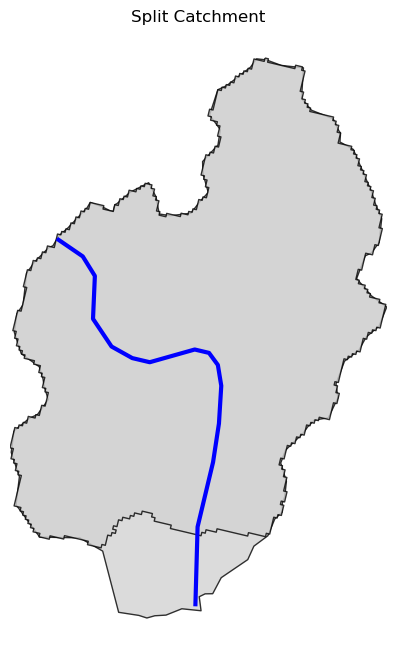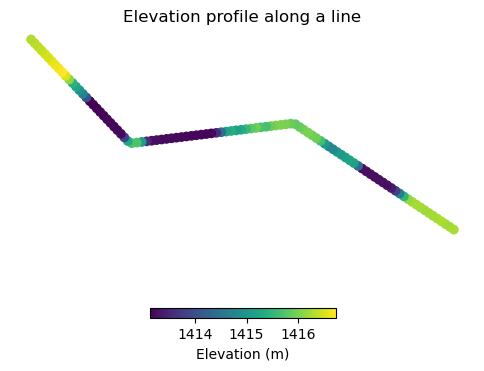This page was generated from pygeoapi.ipynb. Interactive online version:# Split Catchment with PyGeoAPI#

:

import geopandas as gpd
import shapely.geometry as sgeom

import pynhd


PyGeoAPI service provides four functionalities:

1. flow_trace: Trace flow from a starting point to up/downstream direction.

2. split_catchment: Split the local catchment of a point of interest at the point’s location.

3. elevation_profile: Extract elevation profile along a flow path between two points.

4. cross_section: Extract cross-section at a point of interest along a flow line.

The pygeoapi function in PyNHD requires two inputs, a geopandas.GeoDataFrame that must contain all the required inputs corresponding to target services. Let’s take a look at them in an example:

:

gdf = gpd.GeoDataFrame(
{
"direction": [
"none",
]
},
geometry=[sgeom.Point((1774209.63, 856381.68))],
crs="ESRI:102003",
)
trace = pynhd.pygeoapi(gdf, "flow_trace")


In the split_catchment service we can set upstream to True to get the entire upstream catchments not just the split local catchments.

:

gdf = gpd.GeoDataFrame(
{
"upstream": [
False,
]
},
geometry=[sgeom.Point((-73.82705, 43.29139))],
crs="epsg:4326",
)
split = pynhd.pygeoapi(gdf, "split_catchment")

:

ax = split.plot(figsize=(8, 8), facecolor="lightgrey", edgecolor="black", alpha=0.8)
trace.plot(ax=ax, color="b", linewidth=3.0)
ax.axis("off")
ax.set_title("Split Catchment")
ax.margins(x=0)
ax.figure.set_dpi(100)
ax.figure.savefig("_static/split_catchment.png", bbox_inches="tight", facecolor="w", dpi=100)The elevation_profile service returns the elevation profile along a flow path between two points at a given number of points and a specified resolution for DEM.

:

coords = [(-103.801086, 40.26772), (-103.80097, 40.270568)]
gdf = gpd.GeoDataFrame(
{
"numpts": [
101,
],
"dem_res": [
1,
],
},
geometry=[sgeom.MultiPoint(coords)],
crs="epsg:4326",
)
profile = pynhd.pygeoapi(gdf, "elevation_profile")

:

ax = profile[["distance", "elevation"]].plot(
x="distance", y="elevation", color="b", linewidth=3.0, figsize=(12, 4)
)
ax.set_ylim(1280, 1320)
ax.legend(["Elevation Profile"])
ax.margins(x=0)The cross_section service extracts the cross-section within a buffer distance from a point of interest along a flow line and at a given number of points.

:

gdf = gpd.GeoDataFrame(
{
"numpts": [
101,
],
"width": [
1000,
],
},
geometry=[sgeom.Point((-103.80119, 40.2684))],
crs="epsg:4326",
)
section = pynhd.pygeoapi(gdf, "cross_section")

:

ax = section[["distance", "elevation"]].plot(
x="distance", y="elevation", color="b", linewidth=3.0, figsize=(10, 5)
)
ax.legend(["Cross Section"])
ax.margins(x=0)